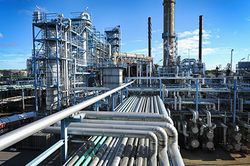# Documentation:CHBE Exam Wiki/Midterm Exam 1 2016W/MT1 Question 2

CHBE 241
Exam resources wikiChemical and Biological Engineering
Welcome to the CHBE Exam Resources Wiki!

This wiki is intended to host past exams

with fully worked-out hints and solutions
Past Exams
Final Exam 2016W
Midterm Exam 1 2016W
Midterm Exam 2 2016W
Problem Sets
Module 1 - Process Basics
Module 2 - Reactors
Module 3 - Separations 1
Module 4 - Separations 2
Module 5 - Non-reactive Energy Balances
Module 6 - Reactive Energy Balances

Gypsum, a solid mineral, is used in the production of drywall in Vancouver. The gypsum entering the plant contains gypsum and 2.00 wt % water and is simultaneously crushed and dried in a hammer mill. The gypsum is dried in the hammer mill using a pure air stream containing no water. Two streams exit the hammer mill, one solid and one gas. The solid stream contains 0.50 wt% water and the remainder as gypsum. The gas stream contains all the air entering, the removed water and 1.00 wt% of gypsum, as fine particles.

In order to recover the gypsum in the air, and remove the fine particles, the gas stream exiting the hammer mill is sent to a cyclone unit. Two streams emerge from the cyclone, one stream of solids containing only gypsum, with 72.0 percent (by weight) of the gypsum particles entering, and the other stream of gas containing all the air and water entering and the remaining gypsum particles. The gas stream exiting the cyclone is sent for further processing elsewhere in the plant. The gypsum recovered in the solid stream exiting the cyclone is mixed in a mixer unit with the solids stream which left the hammer mill. The combination of these solids streams is then sent for further processing elsewhere in the plant.

## Question 2a [20 points]

Draw a process flowchart containing 3 units, labeling all streams with known and unknown variables. List the extra relationships between variables that are known.

## Question 2b [20 points]

Assuming a basis of 50.0 metric tonnes a day of gypsum entering the plant, perform a degree of freedom analysis on each individual unit as well as the overall process.

## Question 2c [15 points]

A pressure gauge reads the pressure of the gas stream entering the cyclone as 10.30 psig (psi gauge). A manometer is also connected between the gas streams entering and leaving the cyclone unit showing a height difference of 20.0 cm. It contains a fluid with a specific gravity of 6.45. The pressure is expected to drop as the gas flows through the cyclone. If the absolute atmospheric pressure is 100 kPa, what is the pressure of the gas stream leaving the cyclone in absolute pressure in kPa?

## Question 2d [5 points]

To calculate the centrifugal force on particles in the cyclone, the below equation is used. Show whether the equation is dimensionally consistent. You should know the dimensions, or be able to find the dimensions, for the variables listed.

$F_{C}={\frac {4}{3}}\cdot \pi \cdot \rho _{p}\cdot {r_{p}}^{3}\cdot {u_{t}}^{2}$where
$F_{C}={\text{Centrifugal force}}$$\rho _{p}={\text{particle density}}$$r_{p}={\text{particle radius}}$$u_{t}={\text{tangential velocity}}$Script error: The function "navbox" does not exist.